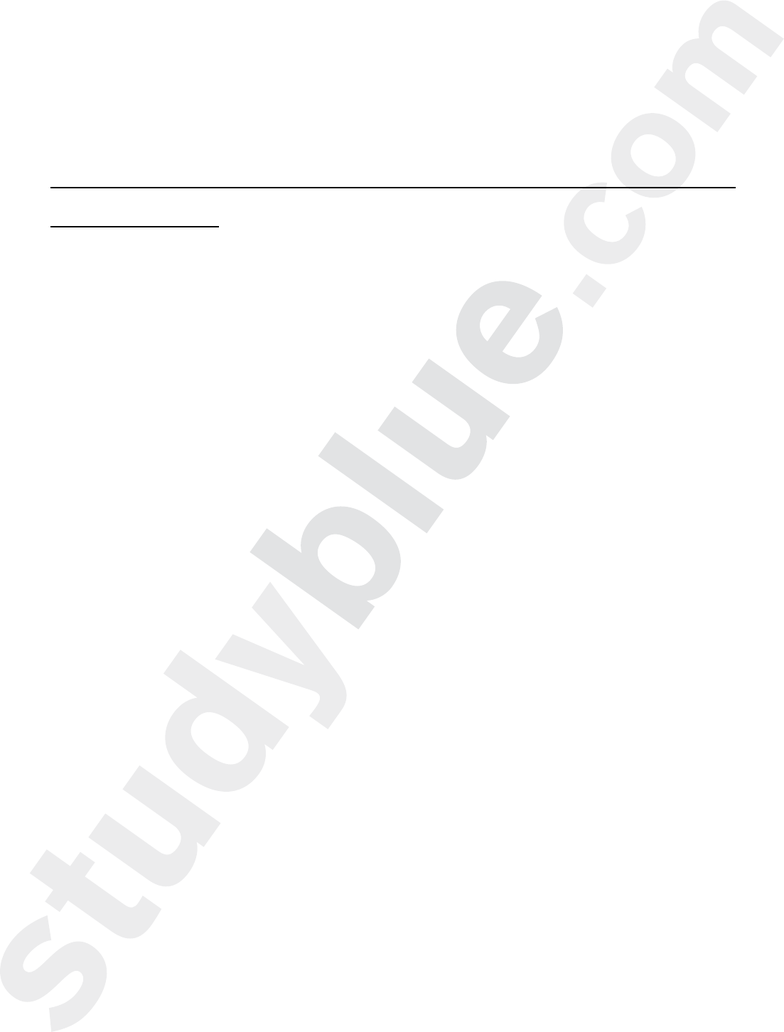Study Guides (400,000)
US (230,000)
Rutgers (3,000)
3:40 (100)
All (30)
Final

# 01:160:161 Study Guide - Final Guide: International System Of Units, Nanometre, Horse Length

This preview shows pages 1-3. to view the full 53 pages of the document.1
CHEM 161-2007
CHAPTER 1 CHEMISTRY: MATTER AND MEASUREMENT
PRACTICE PROBLEMS
DR. ED TAVSS
Units/unit conversions/dimensional analysis
Classification of matter
Significant Figures/Precision/Accuracy
Miscellaneous

Only pages 1-3 are available for preview. Some parts have been intentionally blurred.2
UNITS/UNIT CONVERSIONS/DIMENSIONAL
ANALYSIS
Chem 161-2007 Exam I
Hill, Petrucci et al., 4th edition
Chapter 1 Chemistry: Matter and Measurement
Units/unit conversions/dimensional analysis
4. Which of the following is not a base unit of the SI measurement system?
A. m
B. s
C. kg
D. A
E. atm
m (meters), s (seconds), kg (kilograms), and A (amperes) are basic SI units.
Atm is not an SI unit; the SI unit for pressure is newtons per meter squared (N/m2) and is called the Pascal
(Pa). One atmosphere is 101.325 Pa.
Chem 161-2007 Exam I
Hill, Petrucci et al., 4th edition
Chapter 1 Chemistry: Matter and Measurement
Units/unit conversions/dimensional analysis
15. In the SI metric system, which power of ten is associated with the prefix n?
A. 109
B. 10-6
C. 10-9
D. 106
E. 10-12
“n” means “nano” which means “10-9”; e.g., a nanometer is 1 x 10-9 m.

Only pages 1-3 are available for preview. Some parts have been intentionally blurred.3
Chem 161-2007 Exam I
Hill, Petrucci et al., 4th edition
Chapter 1 Chemistry: Matter and Measurement
Units/unit conversions/dimensional analysis
24. What temperature is numerically the same in both oC and oF?
A. 0
B. 273
C. -40
D. 212
E. -80
oC = 5/9(oF 32)
A. oC = 5/9(oF 32)
oC = 5/9(o0 32) = -18oC
0o -18o
B. oC = 5/9(oF 32)
oC = 5/9(273 32) = 134oC
273o 134o
C. oC = 5/9(oF 32)
oC = 5/9(-40 32) = -40oC
-40o = -40o
D. oC = 5/9(oF 32)
oC = 5/9(212 32) = 100oC
212o 100o
E. oC = 5/9(oF 32)
oC = 5/9(-80 32) = -62oC
-80o -62o
Chem 161-2007 Exam I
Hill, Petrucci et al., 4th edition
Chapter 1 Chemistry: Matter and Measurement
Units/unit conversions/dimensional analysis
13. What is the volume, in L, of a box that is 8.00 in on a side? 1 in = 2.54 cm exactly
A. 8.39
B. 1.30
C. 31.2